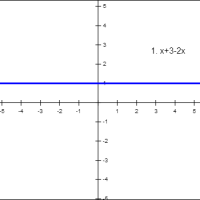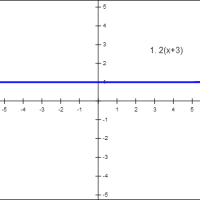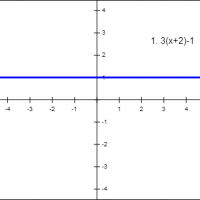# Saltire Software

## Common Core Nuggets :: Equivalent Expressions

This collection contains apps that will help teachers implement Common Core Standard 7.EE.1 for 7th grade mathematics.#### Combining Like Terms I

This app allows you to practice addition and subtraction of linear monomial expressions with rational coefficients.#### Combining Like Terms II

This app allows you to practice addition and subtraction of linear binomial expressions with rational coefficients.#### Distributive Property

In this app you will use distributive property to factor and expand linear expressions with rational coefficients.#### Simplifying Expressions

In this app you will use properties of addition and multiplication to simplify linear expressions with rational coefficients.